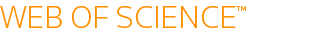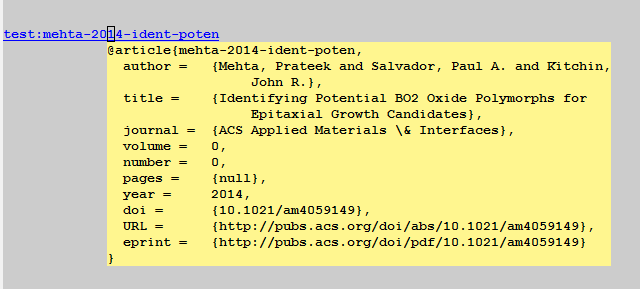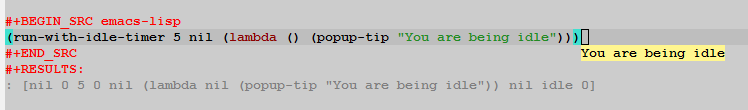## Integration of the heat capacity

| categories: uncategorized | tags: | View Comments

From thermodynamics, the heat capacity is defined as $$C_p = \left(\frac{dH}{dT}\right)_P$$. That means we can calculate the heat required to change the temperature of some material from the following integral:

$$H_2 - H_1 = Q = \int_{T_1}^{T_2} C_p(T) dT$$

In the range of 298-1200K, the heat capacity of CO2 is given by a Shomate polynomial:

$$C_p(t) = A + B t + C t^2 + D t^3 + E/t^2$$ with units of J/mol/K.

where $$t = T / 1000$$, and $$T$$ is the temperature in K. The constants in the equation are

value
A 24.99735
B 55.18696
C -33.69137
D 7.948387
E -0.136638
F -403.6075
G 228.2431
H -393.5224

## 1 Integrate the heat capacity

Use this information to compute the energy (Q in kJ/mol) required to raise the temperature of CO2 from 300K to 600K. You should use scipy.integrate.quad to perform the integration.

### 1.1 solution   solution

A =  24.99735
B =  55.18696
C = -33.69137
D =  7.948387
E = -0.136638
F = -403.6075
G =  228.2431
H = -393.5224

def Cp(T):
t = T / 1000
return A + B*t + C*t**2 + D*t**3 + E / t**2

dH, _ = quad(Cp, 300, 600)
print(f'The change in enthalpy is {dH / 1000:1.3f} kJ/mol')

The change in enthalpy is 12.841 kJ/mol



## 2 Verify via Δ H

The change in enthalpy (in kJ / mol) from standard state is

$$dH − dH_{298.15}= A t + B t^2/2 + C t^3/3 + D t^4/4 − E/t + F − H$$

again, $$t = T / 1000$$.

Use this equation to compute the change in enthalpy when you increase the temperature from 300 K to 600 K.

### 2.1 solution   solution

def dH(T):
t = T / 1000
return A * t + B*t**2 / 2 + C * t**3 / 3 + D * t**4 / 4 - E/t + F - H

print(f'The change in enthalpy is {dH(600) - dH(300):1.3f} kJ/mol')

The change in enthalpy is 12.841 kJ/mol



org-mode source

Org-mode version = 9.1.13

## helm actions when there is no match

| categories: uncategorized | tags: | View Comments

Sometimes you run out of matches in a helm selection buffer, and all that is left is the pattern you have typed in. It turns out you can perform some action on that pattern! Why would you do that? Suppose you are searching your bibliography, and you do not find what you are looking for. Then, you may want to send the pattern to Google, or some other search engine to see what comes up.

The key to handling this situation is to use two sources in your helm session. One that works on the candidates and deals with actions on them, and one that has no candidates, and works on the pattern. The variable helm-pattern contains what you typed in. We call the second source the Fallback option. The second source has no candidates, and we use (dummy) in place of the candidates.

It easy to add two sources. Here we define the sources as variables, and use the variables in the :sources list to the helm command.

(defun some-action (arg)
(message-box "%s\n%s"
(helm-get-selection)
(helm-marked-candidates)))

(defun default-action (candidate)
(browse-url
(format

(defvar source1 '((name . "HELM")
(candidates . (1 2 3 4))
(action . (("open" . some-action)))))

(defvar fallback-source '((name . "fallback")
(dummy)

(helm :sources '(source1 fallback-source))

#<process open http://www.google.com/search?q=addtion%20pul>


When you run this, if you run out of search candidates, all that will be left is the fallback option, and when you press enter, it will launch a browser pointing to the google search for your pattern.

org-mode source

Org-mode version = 8.2.10

## New org-mode link to Web of Science

| categories: uncategorized | tags: | View Comments

For ages I have been trying to figure out how to make a link to open a search in Web of Science. Today, thanks to help from our library, I finally figured it out!

It turns out you can embed a search widget to Web of Science in a web page. See http://wokinfo.com/webtools/searchbox/ . Here is an example.Search Web of Science™     Copyright 2014 Thomson Reuters

This simple form just sends a GET http request to a cgi script at Web of Knowledge. Awesome, we can create a url that does just that to make an org link! We will make a link that you can click on to open the web page, and a simple formatting function to make the link work in html too when we export it.

(org-add-link-type
"wos"
(lambda (path)
(browse-url
(s-join "+"
(split-string path)))))
;; formatting function. Assume html
(format "<a href=\"%s\">%s</a>"
(s-join "+"
(split-string path)))
)))


Now, here is a link: wos:alloy segregation

When I click on it in org-mode, Web of Science opens to articles that match that search. When I export the post to html, you should also see a link that opens to Web of Science (assuming you click on it from an IP address with access).

The link may not seem all that useful, but we can use the idea to highlight words, and send them to a web of science query, e.g. https://github.com/jkitchin/jmax/blob/master/words.el#L63 , or in org-ref to query web of science for the words you typed into helm-bibtex that do not match any references in your database. One more powerful tool in doing research for a living!

org-mode source

Org-mode version = 8.2.10

## Pandoc does org-mode now

| categories: uncategorized | tags: | View Comments

Pandoc (http://johnmacfarlane.net/pandoc/ ) is a document converter. It does a pretty good job of converting a document in one format to another. Pandoc also knows about org-mode now, and can convert an org-file to a Word document! We are going to test it out in this post to see what it does well with.

## 1 A subsection with some equations

Einstein showed us that $$E = mc^2$$.

A matrix looks like this:

\begin{equation} \begin{matrix} a & b & c \\ d & e & f \\ g & h & i \end{matrix} \end{equation}

## 2 A section with a figure

Here is a figure in the document.Figure 1: A cosine function.

## 3 A section with a table

Table 1: A simple table.
x y
1 1
2 4
3 9

## 4 Some citations

For fun, a reference to the org-mode book dominik-2010-org-mode.

## 5 some source code

here is a python block.

print 'hello pandoc'

hello pandoc


and finally, we write a block that will convert this file to a word document.

(save-buffer)
(shell-command "pandoc -s -s org-to-word.org -o org-to-word.docx")

0


Now, here is that org-to-word.docx

it is pretty good, and blazing fast. The output is not quite as good as the native org to pdf (org-to-word.pdf ), but since the translation is happening outside of Emacs the results are still pretty impressive, and if you need a Word document there is no substitute 1. The simple equation was translated to a Word equation format (cool!) but the matrix did not show up in the word document, nor did the figure caption. The code does show up, but the lines are not numbered as they are in the pdf. The citation did not work out of the box. The User guide suggests it might be possible to get this to work with a citations extension though.

I am impressed that the Word document has proper section headings. Overall, my impression is that this is a very good way to get 90+% of the way to a finished word document with an org-source file!

## Footnotes:

1

Ok, there is the ODT export engine. So far I have not been able to make that export documents that Word can open though, and it takes more configuration than just installing Pandoc.

org-mode source

Org-mode version = 8.2.6

## Popup tips on bibtex links in org-mode

| categories: uncategorized | tags: | View Comments

I want to explore using popup tips to display richer information about org-mode links. The idea is to have something like a tooltip that displays the bibtex entry when you hover over it, or click on it.

Here is a canonical example of a popup.

(popup-tip "Hello, World!")

tAll I need to do is figure out a simple way to get the bibtex entry as a string, and pop it up when a link is clicked on.

(org-add-link-type
"test"
;; this function is run when you click
;; formatting
(lambda (keyword desc format)
(cond
((eq format 'html) (format "<pre>%s:%s</pre>" keyword desc)))))

 lambda (link-string) (popup-tip link-string) lambda (keyword desc format) (cond ((eq format (quote html)) (format
%s:%s
keyword desc)))

Now we give it a try.

test:show-me-the-popupThat looks good.

Ok, the penultimate step will be to lookup a bibtex entry, and show the entry in a popup. We will hardcode the path to the bibtex file.

(org-add-link-type
"test"
;; this function is run when you click
(lambda (bibtex-key)
(let ((entry (with-temp-buffer
(insert-file-contents "~/Dropbox/bibliography/references.bib")
(goto-char (point-min))
(re-search-forward bibtex-key)
(bibtex-narrow-to-entry)
(buffer-string))))
(popup-tip entry))))

 lambda (bibtex-key) (let ((cb (current-buffer)) (entry (with-temp-buffer (insert-file-contents ~/Dropbox/bibliography/references.bib) (goto-char (point-min)) (re-search-forward bibtex-key) (bibtex-narrow-to-entry) (buffer-string)))) (popup-tip entry))

test:mehta-2014-ident-poten

And here is what appears for me:The final step is to connect this to an idle timer . We want a popup to occur when our mouse is idle. I am setting this up to run one time, after 5 seconds of idleness.

(run-with-idle-timer 5 nil (lambda () (popup-tip "You are being idle")))

[nil 0 5 0 nil (lambda nil (popup-tip "You are being idle")) nil idle 0]So, we need to setup an idle timer that runs on some interval. When the cursor is on the right kind of link, we want to get a popup. I adapted the following code from http://www.emacswiki.org/emacs/IdleTimers .

;; variable for the timer object
(defvar idle-timer-bibtex-timer nil)

;; callback function
(defun idle-timer-bibtex-callback ()
"displays a popup of the bibtex entry in a test link"
(interactive)
(let ((object (org-element-context)))
(when (and (equal (org-element-type object) 'link)
(equal (org-element-property :type object) "test"))
(let* ((bibtex-key (org-element-property :path object))
(entry (with-temp-buffer
(insert-file-contents "~/Dropbox/bibliography/references.bib")
(goto-char (point-min))
(re-search-forward bibtex-key)
(bibtex-narrow-to-entry)
(buffer-string))))
(popup-tip entry)))))

;; start functions
(defun idle-timer-bibtex-start ()
(interactive)
(when (timerp idle-timer-bibtex-timer)
(cancel-timer idle-timer-bibtex-timer))
(setq idle-timer-bibtex-timer
(run-with-timer 1 1 #'idle-timer-bibtex-callback)))

;; stop function
(defun idle-timer-bibtex-stop ()
(interactive)
(when (timerp idle-timer-bibtex-timer)
(cancel-timer idle-timer-bibtex-timer))
(setq idle-timer-bibtex-timer nil))

(idle-timer-bibtex-start)

idle-timer-bibtex-stop


test:kitchin-2008-alloy

Now, whenever the cursor is on the link, and there is an idle of about a sec, I get a popup window of the bibtex entry. It looks like this:There are still some limitations to this code. It does not handle multiple citations in a link (like the cite links I normally use do). That will take a little work to fixup. I cannot figure out how to get mouse-over tooltips; this only works when the cursor is on the link. I do not know what the optimal timer setting is. This one runs every second. I do not see any issues in performance with that. Another issue might be making the timer a file local variable. It would be nice if the timer quit running when the file was closed. I do not know how easy that would be to implement, or if there should be one timer running for org-mode. Finally, this code is hard-coded to use my reference file. For a real module, we would probably provide some customization to choose other bibtex files. Overall though, this might be a handy way to quickly peruse the citations in an org-file.